Home > CCA2 > Chapter 8 > Lesson 8.1.1 > Problem8-16

8-16.
1. Without a calculator, find two solutions 0° ≤ θ < 360° that make each of the following equations true. Homework Help ✎1. cos θ =2. tan θ = −1

3. sin θ =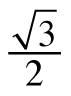4. cos θ = −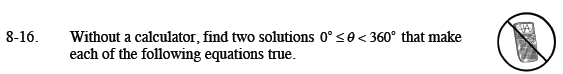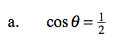Draw a unit circle.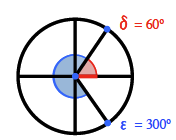$\text{The cosine is where the }x\text{-value (red line) is }\frac{1}{2}.$

Answers: 60° and 300°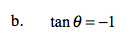135° and 315°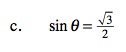Draw and label a unit circle as in part (a). Don't forget that the sine is the y-value! Which quadrants will the angles be in?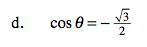See part (c).